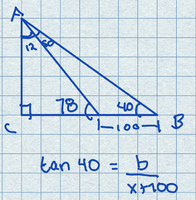# The height of a building.

#### Dirty.Rotten.Imbecile

##### New member
At a certain distance from a 20 storey building, you measure the angle of elevation to the 10th floor to be 40 Deg. After walking 100 m closer to the building, you measure the angle of elevation to the same 10th floor to be 78 deg. How high is the 20th storey?

I drew a diagram it I have two variables in the resulting equation so I am not sure where to go with it. This problem is for a practice test but the unit never covers a problem like this at all.#### onesun0000

##### New member
At a certain distance from a 20 storey building, you measure the angle of elevation to the 10th floor to be 40 Deg. After walking 100 m closer to the building, you measure the angle of elevation to the same 10th floor to be 78 deg. How high is the 20th storey?

I drew a diagram it I have two variables in the resulting equation so I am not sure where to go with it. This problem is for a practice test but the unit never covers a problem like this at all.

View attachment 12971
You can use the Law of Sines to solve for the length of hypotenuse AB. Try to figure out the rest of the steps, then post your solution so we can check.

•Dirty.Rotten.Imbecile

#### Otis

##### Senior Member
… I have two variables in the resulting equation so I am not sure where to go with it …
Hello. You wrote a good equation, and, yes, it contains two unknowns:

tan(40º) = b/(x + 100)

This suggests that we need a second equation (solving for 2 variables requires 2 equations). Use the other triangle to write a second equation with symbols x and b.

tan(78º) = b/x

Now you have a system of two equations. Solve it for x and b.

Remember: symbol b represents the height to the 10th floor, but the question asks for the height to the 20th floor.•Dirty.Rotten.Imbecile

#### Dr.Peterson

##### Elite Member
I'm very much hoping that the 10's and 20's are really meant to be all the same number. We really know nothing at all about the 20th storey. Even if we knew (a) that all storeys are the same height, rather than the ground floor being taller as often happens; (b) that the building doesn't skip the 13th, as some do; and (c) whether the measurement was made to the top of the 10th, or the bottom, or a point in the middle, and likewise for the 20th -- a lot depends on whether we say that the first storey is the ground floor or the first one up. (I suspect that people who call them "storeys" use the latter convention, which does fit better with math.)

I can't believe that the author would have mixed that kind of trickery into a trig problem!

•Dirty.Rotten.Imbecile

#### Dirty.Rotten.Imbecile

##### New member
Thanks for the help everyone.

#### Otis

##### Senior Member
Hi D.R.I. Your solution for x matches mine (we each rounded to four places), and my final answer (approximately 204.25 meters) is very close to yours. (I didn't check all of your work.)

Here's what I did, after solving for x. Each of us had already written the formula for b in terms of x:

b = 4.7046x

So I evaluated b as (4.7046)(21.7074), and then doubled it (rounding arbitrarily, to two places).

2b = (2)(102.1246) = 204.2492

It might be better for each of us to report "approximately 204 m", unless you were told to round otherwise.#### Dirty.Rotten.Imbecile

##### New member
Yes I’ve noticed this with several solutions in the text I am working with. Your answer makes sense.
On my calculator, dividing 78.2335 by 0.766 = 102.1325. 102.1325(2) = 204.265 which also seems to make sense.
And the actual solution from the text is 204.26

At the start of the test it says to round all angles to the nearest degree and all distances and lengths to two decimal places.

I am not sure how they will ultimately grade me on the real test. I intended to ask about it.

#### Otis

##### Senior Member
… the actual solution from the text is 204.26 …
Their solution is off by a centimeter, due to rounding intermediate results (known as 'round-off error').

… it says to round … lengths to two decimal places …
That instruction pertains to final answers only. Were we to round intermediate lengths to two places, the round-off error would increase.

Your answer is off by 2 cm because of round-off error. You can avoid that, by rounding every intermediate value to five places instead of four.

I would have expected that rounding intermediate results to four places would have been sufficient (it was, using my approach to estimate b), but you calculated b via two additional sine values, and the rounding of those sines is what changed your final answer a tiny bit. Alternatively, you could round the lengths to four places and not round any trigonometric values displayed by the calculator (that is, let the calculator carry all digits for subsequent calculations, by using its memory features).

Assuming that round-off error has not been covered in your class, your work is good. I would have given you full credit.#### Dirty.Rotten.Imbecile

##### New member
Thanks!

My calculator is set to round at four decimals so that explains a lot!